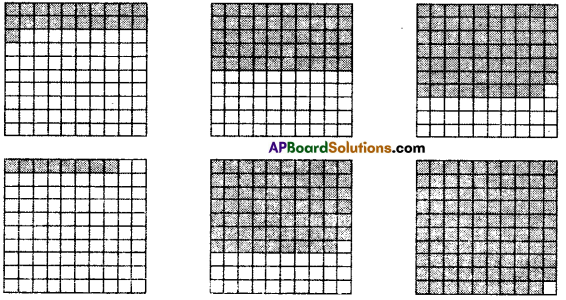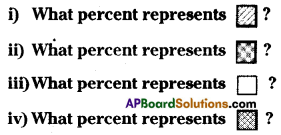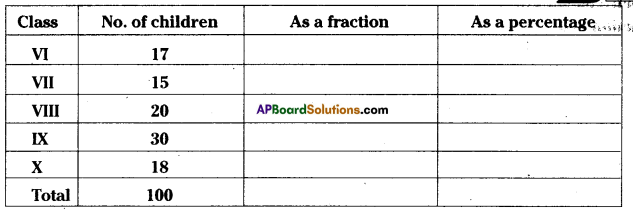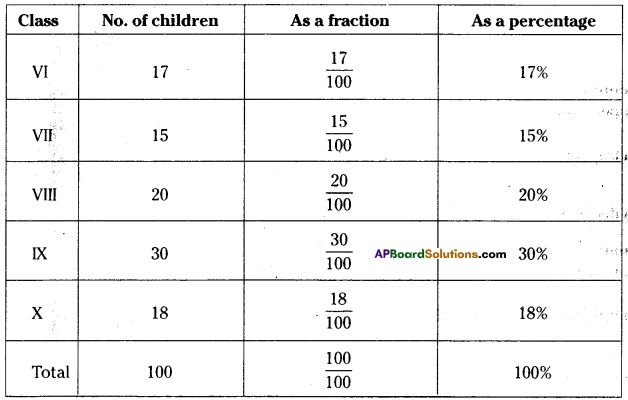# AP Board 7th Class Maths Solutions Chapter 6 Ratio – Applications InText Questions

AP State Syllabus AP Board 7th Class Maths Solutions Chapter 6 Ratio – Applications InText Questions and Answers.

## AP State Syllabus 7th Class Maths Solutions 6th Lesson Ratio – Applications InText QuestionsTry This

Question
Think of some real life situations in which you have to compare quantities in the form of a ratio. (Page No. 111)
Solution:

1. Boys and girls ratio in a class.
2. Number of matches won and lost by India at World Cup.
3. Number of students come by bycycle and on foot.Do This

Question 1.
40 benches are required to seat 160 Sfciidents. How many benches will be required to seat 240 students at the same rate ?  (Page No. 116)
Solution:
Number of benches required to seat 160 students= 40
On one bench, the number of students that can be seated = $$\frac{160}{40}$$ = 4 . 40
∴ For 240 students the number of benches required = $$\frac{240}{4}$$= 60

Question 2.
When a Robin bird flies, it flaps wings 23 times in 10 sec. How many times will it flap its wings in 2 minutes ? (Page No. 116)
Solution:
In 10 sec. Robin bird flaps for 23 times.
In 1 sec Robin bird flaps $$\frac{23}{10}$$ times
In 2 minutes = 2 x 60 = 120 sec Robin bird flaps for = $$\frac{23}{10}$$ x 120 = 276 timesQuestion 3.
The average human heart beats at 72 times per minute. How maiiy times does it beat in 15 seconds ? How many in 1 hour ? How many in a day ? (Page No. 116)
Solution:
Number of beats per 1 minute (60 seconds) = 72 times
Number of beats per 1 second = $$\frac{72}{60}=\frac{6}{5}$$ times
Number of beats per 15 seconds = $$\frac{6}{5}$$ x 15 =18 times
∴ Number of beats per 1 hour = $$\frac{6}{5}$$ x 60 x 60 = 4320 times
∴ Number of beats per 1 day = 4320 x 24 = 103680 times.

Try This

Question 1.
Population of our country as per 2011 census is about 12 x 108 (120,00,00,000). If the popu-lation of our country increases by 3% every year what will be the population by 2012 ? (Page No. 126)
Solution:
Population in 2011 = 12 x 108
It increases by 3%
Population in 2012 = (100 + 3) % of 2011
= 103% of 12 x 108
= $$\frac{103 \times 12 \times 10^{8}}{100}$$
= $$\frac{1236 \times 10^{8}}{100}$$
= 12.36 x 108 .Question 2.
i) Can you eat 75% of a dosa ? (Page No. 126)
Solution:
Yes.

ii) Can the price of an item go up by 90% ?
Solution:
Yes.

iii) Can the price of an item go up by 100% ?
Solution:
Yes.Do This

Question 1.
Find the interestonasum of825O for3yearsat the rate of 8%perannum. (Page No. 138)
P = ₹ 8250 R = 8% T = 3 years
Interest =P x R% x T
I = 8250 x $$\frac{8}{100}$$ x 3 .
Interest = $$\frac{198000}{100}$$ = 1980

Question 2.
₹ 3000 is lent out at 9% rate of interest. Find the Interest which will be reèieved at the end of 2½ years. (Page No. 138)
Sol. P = ₹3000 R = 9% T = 2½ years = $$\frac{5}{2}$$,
Interest = P x R% x T
3000 x $$\frac{9}{100} \times \frac{5}{2}$$ = ₹675

Do This

Question
Given below are various grids of 100 squares. Each has a different number of squares coloured.
In each case, write the coloured and white part in the form of a 1) Percentage 2) FractIon and 3) Decimal (Page No. 121)Solution:
I) Coloured squares = 21
as a percentage 21%
as a fraction = $$\frac{21}{100}$$
asadecimal = $$\frac{21}{100}$$ =0.21

ii) Coloured squares = 50
as a percentage = 50%
asafraction = $$\frac{50}{100}=\frac{1}{2}$$
as a decimal = $$\frac{50}{100}$$ = 0.50iii) Coloured squares = 69
as a percentage = 69%
as a fraction = $$\frac{69}{100}$$
as a decimal = $$\frac{69}{100}$$ = 0.69

iv) Coloured squares = 8
as a percentage = 8%
as a fraction = $$\frac{8}{100}=\frac{2}{25}$$
as a decimal = $$\frac{8}{100}$$ = 0.08

v) Coloured squares = 70
as a percentage = 70%
as a fraction = $$\frac{70}{100}$$
as a decimal = $$\frac{70}{100}$$ = 0.70

vi) Coloured squares = 99
as a percentage = 99%
as a fraction = $$\frac{99}{100}$$
as a decimal =$$\frac{99}{100}$$ = 0.99

Question 2.
Look at the grid paper given below. It Is shaded In various designs. Find the percentage of each design (Page No. 122)Solution:
(i) 19%
(ii) 40%
(iii) 34%
(iv) 7%Question 3.
The strength particular of a school are given below. Express the strength of each class as a fraction, percentage of total strength of the school. (Page No.122)Solution:Try This

Question 1.
The C.P of 12 mangoes is equal to the selling price (S.P) of 15 mangoes. Find the loss percent. (Page No. 128)
Solution:
C.P. of 12 = S.P of 15
∴ Loss = 3
Loss percentage = $$\frac{3}{15}$$ x 100% = 20%